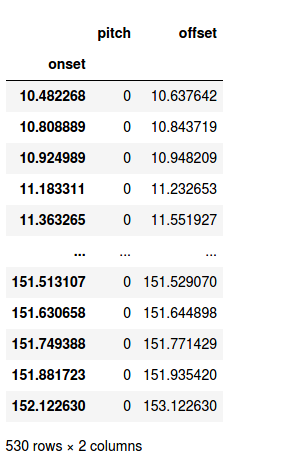# Help with merging dataframes

Hi Pandas experts.

Here’s a dataframe, mel1, indexed by float64 (a time):Here’s a second dataframe, silences, with the same kind of index:I merely want to interleave the two dataframes in order of the onset index. That should not be hard, right? But I have read the docs until my eyeballs are falling out. Can you please help?

1 Like

import pandas as pd

pd.merge lets you do a join by setting how {‘left’, ‘right’, ‘outer’, ‘inner’}. If you want the join to be on the index, left_index and right_index arguements are what you are looking for.

If you just want to make a dataframe that is the length of both dataframes combined (ie insert the second one in the first). pd.concat([df1,df2]) should do that.

If you need to then sort by the index, then https://pandas.pydata.org/pandas-docs/stable/reference/api/pandas.DataFrame.sort_index.html

2 Likes

Hi Enzo. Thanks for responding. pd.concat() duplicated columns when the two dataframes both had column names in common. But df1.append(df2), listed in related functions, combined columns with the same name, just as I wanted. df.sort_index(inplace=True) then interleaved the rows properly.

Thanks again. Malcolm

1 Like